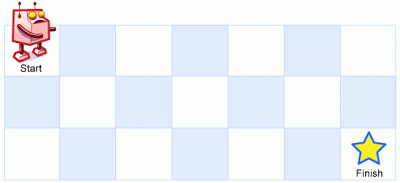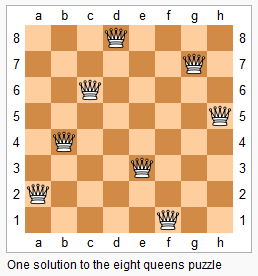## unique path

### 题目描述

A robot is located at the top-left corner of a m x n grid (marked ‘Start’ in the diagram below).The robot can only move either down or right at any point in time. The robot is trying to reach the bottom-right corner of the grid (marked ‘Finish’ in the diagram below).How many possible unique paths are there?Above is a 3 x 7 grid. How many possible unique paths are there?Note: m and n will be at most 100.

### 题目解析

path[i][j]=path[i-1][j]+path[i][j-1];

### 代码实现

 int uniquePaths(int m, int n) { vector<vector<int> >path(m,vector<int >(n,1)); for(int i=1;i<m;i++) for(int j=1;j<n;j++) { path[i][j]=path[i-1][j]+path[i][j-1]; } return path[m-1][n-1]; }

## n queens ii

### 题目描述

Follow up for N-Queens problem.Now, instead outputting board configurations, return the total number of distinct solutions.### 题目解析

void Backtrack(int t,int num) { if(t>num) { countn++; } else for(int i=1;i<=num;i++) { x[t]=i; if(Place(t)) Backtrack(t+1,num); } }void Backtrack(int t,int num) { if(t>num) { countn++; } else for(int i=1;i<=num;i++) { x[t]=i; if(Place(t)) Backtrack(t+1,num); } }

bool Place(int t) { bool ok=true; for(int j=1;j<t;j++) { if(x[t]==x[j]||t-j==fabs(x[t]-x[j]))//判断列对角线是否冲突 { ok=false; break; } } return ok; }

### 代码实现

public: int countn=0; int x; bool Place(int t) { bool ok=true; for(int j=1;j<t;j++) { if(x[t]==x[j]||t-j==fabs(x[t]-x[j]))//判断列对角线是否冲突 { ok=false; break; } } return ok; } void Backtrack(int t,int num) { if(t>num) { countn++; } else for(int i=1;i<=num;i++) { x[t]=i; if(Place(t)) Backtrack(t+1,num); } } int totalNQueens(int n) { Backtrack(1,n); return countn; }

## merge sorted array

### 题目描述

Given two sorted integer arrays A and B, merge B into A as one sorted array.Note:
You may assume that A has enough space to hold additional elements from B. The number of elements initialized in A and B are mand n respectively.

### 题目解析

x1>=0 && x2>=0

A[index--] = A[x1] > B[x2] ? A[x1--] : B[x2--];

if(A[x1]>B[x2]){ A[index]=A[x1]; --index; --x1;}else{ A[index]=B[x2]; --index; --x2;}

• 原来的A和B都已经完整插入到A中，即x1=-1且x2=-1。
• 原来B已经插入完毕了但是A还没有，即x2=-1且x1>=0，此时A剩下的数字都在正确的位置，不需要再排序了。
• 原来的A插入完毕了，B还没有，即x1=-1且x2>=0，那就直接把B插入到剩下的位置即可。

### 代码实现

void merge(int A[], int m, int B[], int n) { int total = m + n; int x1 = m - 1; int x2 = n - 1; int index = total - 1; while (x1 >= 0 && x2 >= 0) { A[index--] = A[x1] > B[x2] ? A[x1--] : B[x2--]; } while (x2 >= 0) A[index--] = B[x2--]; }